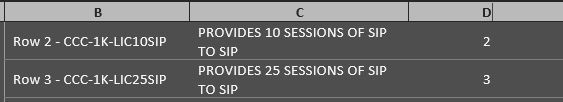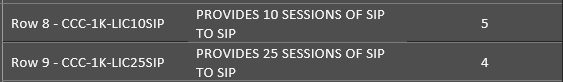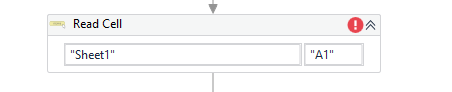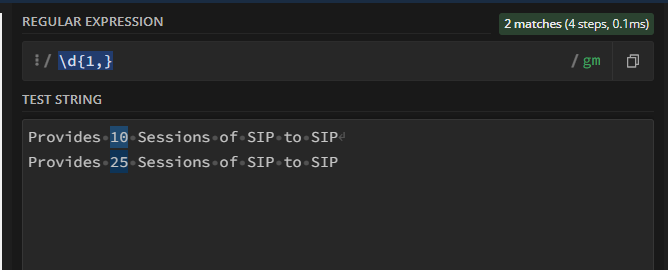# Complex Filter Datatable Question - Process All Rows Pertaining to 1 Cell in 1 Column

Greetings,

I hope my subject line is conveying my question correctly.

I imagine the following would possibly be used:

• Regex
• Filter Datatable
• While/Do While

What I am trying to do:
Column A = Number of sites. (In this example, there are obviously 2.)

How do I?

• Filter the data table so rows 2 – 6 are seen as belonging to the top cell in Column A
• Filter the datatable so rows 8 – 12 are seen as belonging to the bottom cell in Column A

For more context/to help explain what I am trying to accomplish: I would then be looking to filter the datatable/use RegEx so that any cells in Columns, B, C, and D that contain:Would take the “10” and “25” from the strings in Rows 2/3 in Column C and then multiply each respective one by the amount in Column D

So:

• 10 x 2 = 20
• 25 x 3 = 75
o Total = 95

It would then save “95” as a variable

Then for the 2nd site,• 10x5 = 50
• 25x4 = 100
o Total = 150, saved as a variable

Please keep in mind, the “Row 1, Row 2, etc” would not be part of the excel spreadsheet – I typed those in for reference.

So, to recap and restate my question: Using the filter datatable/Regex/whatever activity is best…What is the best way to go about making sure that rows 2 – 6 are seen as belonging to the top Column A cell, and rows, and rows 8 – 12 seen as belonging to the bottom Column A cell?

I am ultimately building a process that generates proposals/contracts for a telecom company. I am trying to make it so it can count the number of sites, and then populate the details of each site on the contract.

Thank you so much for any assistance. This forum is incredible.

Hi @Sc100 , I hope you are doing well.
For this: “For more context/to help explain what I am trying to accomplish: I would then be looking to filter the datatable/use RegEx so that any cells in Columns, B, C, and D that contain:”
1)Inside the for each datatable row you have to assign an integer pointer which will continuously but the initial value should be 1 as first row starts with “A2,B2, etc.” increase by 1 with each row in datatable.
2)After that- you can use row(“B”).contains(“CCC-LIC-1K”) and using integer pointer you will get the row number.
3)Now putting that row number along with column i.e. C pass it to Read cell activity which will give you that cell’s value.4)Suppose if row(“B”).contains(“CCC-LIC-1K”) and pointer is 2 the in Read cell activity the cell index to pass will ne “C”+int_pointer.toString but int_pointer=2 so C2 cell’s value will be extracted which is “Provides 10 Sessions of SIP to SIP”.
5)The same will be done for column D add one more Read cell activity which will give D2 value i.e. “2”.
6)Now for C2 value we need to apply regex with expression: \d{1,}now store this extracted value in a variable.
7)Now you can multiply this value with D2 value and save it as a variable too.

Thanks & Regards,
Shubham Dutta

1 Like

two of a few techniques canbe applied:

Fiiling up - Group By

• after read in a datatable we can fillup the missing a values
• then with a group by we can build and process the groups

Index calculation - Skip/Take Segmentation

• calculate all rows index where A col is not empty
• then slice the data with skip and take like
dtVar.AsEnumerable.Skip(0).Take(6-0),dtVar.AsEnumerable.Skip(6).Take(X-6) …

We also would recommend to filter out any blank seperator rows before running any aproach mentioned above

PPR,

Thank you for coming to the rescue, per usual -

I will certainly try out the info you provided, but I also had another idea on how to approach this -

What we try to split the datatable into separate datatables?

Also, to expand upon this idea, please see a larger screenshot of the excel file I am working with:

What if I did:

• Do While until (Column E) contains “Extended List Price”
• Then, Split Datatable
• Continue the next iteration until it reaches “Extended List Price” again

Thank you again for the continued support and assistance!